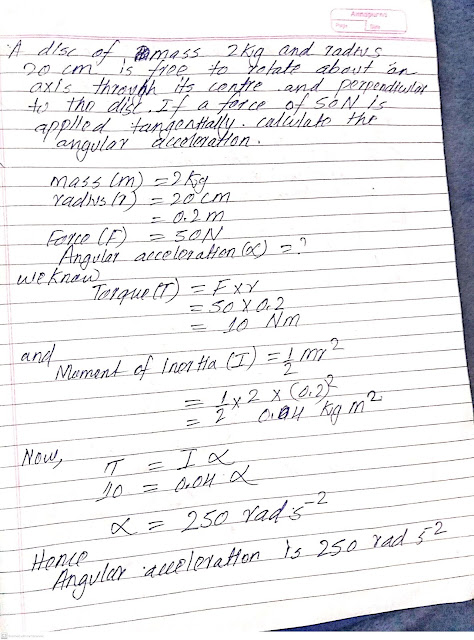# A disc of mass 2kg and radius 20cm is free to rotate about an axis through it centre and perpendicular to the disc.If a force of 50N is applied tangentially. Calculate the angular acceleration.

Image Solution:A disc of mass 2kg and radius 20cm is free to rotate about an axis through it centre and perpendicular to the disc.If a force of 50N is applied tangentially.Calculate the angular acceleration

Related Post: